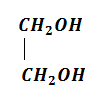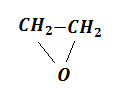# NEET Chemistry Alcohols,Phenols and Ethers Questions Solved

Which of the following will not form a yellow precipitate on heating with an alkaline solution of iodine?

(a) CH3CH(OH)CH3                                  (b) CH3CH2CH(OH)CH3

(c) CH3OH                                               (d) CH3CH2OH

Complete Question Bank + Test Series
Complete Question Bank

Difficulty Level:

n-propyl alcohol and iso-propyl alcohol can be chemically distinguished by which reagent

(a) ${\mathrm{PCl}}_{5}$

(b) reduction

(c) oxidation with potassium dichromate

(d) ozonolysis

Complete Question Bank + Test Series
Complete Question Bank

Difficulty Level:

Among the following sets of reactants which one produces anisole?

(a) CH3CHO, RMgX

(b) C6H5OH, NaOH, CH3I

(c) C6H5OH, neutral FeCl3

(d) C6H5-CH3, CH3COCl, AlCl3

Complete Question Bank + Test Series
Complete Question Bank

Difficulty Level:

Consider the following reaction,

Ethanol$\stackrel{{\mathrm{PBr}}_{3}}{\to }$$\stackrel{\mathrm{Al}.\mathrm{KOH}}{\to }$ Y Z

The product Z is

(a) CH2=CH2

(b) CH3CH2OCH2CH3

(c) CH3CH2OSO3H

(d) CH3CH2OH

Complete Question Bank + Test Series
Complete Question Bank

Difficulty Level:

In the following sequence of reactions,

${\mathrm{CH}}_{3}-\mathrm{Br}\stackrel{\mathrm{KCN}}{\to }$A$\stackrel{{\mathrm{H}}_{3}{\mathrm{O}}^{+}}{\to }$B$\underset{\mathrm{ether}}{\overset{{\mathrm{LiAlH}}_{4}}{\to }}$C

the end product C is

(a) acetone                    (b) methane

(c) acetaldehyde            (d) ethyl alcohol

Complete Question Bank + Test Series
Complete Question Bank

Difficulty Level:

Which one is formed when sodium phenoxide is heated with ethyl iodide?

(a) Phenetole

(b) Ethyl phenyl alcohol

(c) Phenol

(d) None of the above

Complete Question Bank + Test Series
Complete Question Bank

Difficulty Level:

Identity Z in the sequence of reactions,

CH3CH2CH=CH2 $\stackrel{\mathrm{HBr}/{\mathrm{H}}_{2}{\mathrm{O}}_{2}}{\to }$Y$\stackrel{{\mathrm{C}}_{2}{\mathrm{H}}_{5}\mathrm{ONa}}{\to }$Z

(a) CH3-(CH2)3-O-CH2CH3

(b) (CH3)2CH2-O-CH2CH3

(c) CH3(CH2)4-O-CH3

(d) CH3CH2-CH(CH3)-O-CH2CH3

Complete Question Bank + Test Series
Complete Question Bank

Difficulty Level:

Reaction of ethyl formate with excess of CH3MgI followed by hydrolysis gives

(1) n-propyl alcohol                  (2) ethanol              (3) isopropyl alcohol              (4)  propanal

Complete Question Bank + Test Series
Complete Question Bank

Difficulty Level:

Ethylene oxide when treated with Grignard reagent yields

(a) secondary alcohol                                 (b) tertiary alcohol

(c) cyclopropyl alcohol                                (d) primary alcohol

Complete Question Bank + Test Series
Complete Question Bank

Difficulty Level:

In a reaction,

${\mathrm{CH}}_{2}={\mathrm{CH}}_{2}\underset{\mathrm{acid}}{\overset{\mathrm{Hypochlorous}}{\to }}$M$\stackrel{\mathrm{R}}{\to }$M=molecules, R=reagent. M and R are

(a) ${\mathrm{CH}}_{3}{\mathrm{CH}}_{2}\mathrm{Cl}$ and NaOH

(b)  and aq. ${\mathrm{NaHCO}}_{3}$

(c) ${\mathrm{CH}}_{3}{\mathrm{CH}}_{2}\mathrm{OH}$ and HCl

(d)and heat